Chapter 5 – Arithmetic Operations and the ALU

The subject for this chapter is the ALU (Arithmetic Logic Unit) of a typical stored program
computer.  This chapter, by necessity, includes materials on binary addition (half adders, full
adders, and several parallel adders), binary subtraction, binary shifting, and binary multiplication
as implemented by a simplistic algorithm.  In this chapter, we shall reserve the plus symbol “+
for addition and the multiplication symbol “
·” for that operation.

As an extension of the arithmetic operations, we shall consider the basic logical operations of the
ALU.  While some textbooks include a discussion of floating–point arithmetic in a chapter such
as this, we note the common practice of assigning all such operations to a coprocessor.

The first thing to note in this chapter is that we are contemplating a significant leap in our
reasoning, one that normally merits very little mention in most textbooks.  We are planning to
use the tools of Boolean algebra in the service of binary arithmetic.  But arithmetic and Boolean
algebra are completely different topics, built on completely different axiomatic bases.

Despite these significant theoretical differences, there is a mapping between Boolean algebra and
binary arithmetic that allows the use of Boolean digital gates in arithmetic circuits.  We shall
investigate this mapping in the form of truth tables (from Boolean algebra) for two types of

Consider the decimal number 139.  To be precise, this is not a number but a collection of
symbols each used to represent a number.  We know that the digit “1” represents the number 1,
the digit “3” represents the number 3, and the digit “9” represents the number 9.  The association
of the character string “139” with the number 139 is based on positional notation, which states
that 139 = 1·100 + 3·10 + 9·1 = 1·102 + 3·101 + 9·100.

The above example assumes decimal (base 10) notation, which is the notation most commonly
used by humans for representing integers.  In our studies of digital computers, we must consider
not only decimal numbers but also binary (base 2), octal (base 8) and hexadecimal (base 16).  It
is conventional to represent the base of every number system as a decimal number.  Any other
approach would lead to considerable confusion.

In a positional number system, the value of a string of digits is expressed in terms of powers of
the base B.  Consider the four-digit number, denoted in the abstract as D3D2D1D0.  The value of
this number is given by D3·B3 + D2·B2 + D1·B1 + D0·B0.  For example, consider the number
1101.  The value of this number depends on the base of the number system.

In decimal notation, we have 1·103 + 1·102 + 0·101 + 1·100
= 1
·1000 + 1·100 + 0·10 + 1·1 = 1000 + 100 +1 = 110110.

In octal numbers (base 8), we have
11018 = 1
·83 + 1·82 + 0·81 + 1·80 = 1·512 + 1·64 + 0·8 + 1·1  = 57710.

In hexadecimal numbers (base 16), we have
110116 = 1
·163 + 1·162 + 0·161 + 1·160   = 1·4096 + 1·256 + 0·16 + 1·1 = 445310.

In binary numbers (base 2), we have
11012 = 1
·23 + 1·22 + 0·21 + 1·20 = 1·8 + 1·4 + 0·2 + 1·1 = 1310.

To motivate our discussion of binary addition, let us first look at decimal addition.  Consider
the sum 15 + 17 = 32.  In the standard form in which most of us learned addition, the problem
would be stated as follows:

15
+ 17
32

First, note that 5 + 7 = 12.  In order to speak of binary addition, we must revert to a more basic
way to describe 5 + 7; we say that the sum is 2 with a carry-out of 1.  Consider the sum 1 + 1,
which is known to be 2.  However, the correct answer to our simple problem is 32, not 22,
because in computing the sum 1 + 1 we must consider the carry-in digit, here a 1.  With that in
simpler as it does not involve a carry-in.  The following table considers the sum and carry from
A + B.

A   B       Sum    Carry

0    0          0          0              Note the last row where we claim that 1 + 1 yields a

0    1          1          0              sum of zero and a carry of 1.  This is similar to the

1    0          1          0              statement in decimal arithmetic that 5 + 5 yields a

1    1          0          1              sum of 0 and carry of 1 when 5 + 5 = 10.

Remember that when the sum of two numbers equals or exceeds the value of the base of the
numbering system (here 2) that we decrease the sum by the value of the base and generate a
carry.  Here the base of the number system is 2 (decimal), which is 1 + 1, and the sum is 0.
Say “One plus one equals two plus zero: 1 + 1 = 10”.

For us the half-adder is only a step in the understanding of a full-adder, which implements
binary addition when a carry-in is allowed.  We now view the table for the sum A + B, with a
carry-in denoted by C.  One can consider this A + B + C, if that helps.

Full-Adder: A + B with Carry

A      B      C                Sum       Carry

0       0       0                   0             0

0       0       1                   1             0

0       1       0                   1             0

0       1       1                   0             1

1       0       0                   1             0

1       0       1                   0             1

1       1       0                   0             1

1       1       1                   1             1

As an example, we shall consider a number of examples of addition of four-bit binary
numbers.  The problem will first be stated in decimal, then converted to binary, and then
done.  The last problem is introduced for the express purpose of pointing out an error.

We shall see in a minute that four-bit binary numbers can represent decimal numbers in the
range 0 to 15 inclusive.  Here are the problems, first in decimal and then in binary.

1)      6 + 1             0110 + 0001

2)      11 + 1           1011 + 0001

3)      13 + 5           1101 + 0101

0110     1011     1101            In the first sum, we add 1 to an even number.  This
0001     0001     0101                is quite easy to do.  Just change the last 0 to a 1.
0111     1100     0010            Otherwise, we may need to watch the carry bits.

In the second sum, let us proceed from right to left.  1 + 1 = 0 with carry = 1.  The second
column has 1 + 0 with carry-in of 1 = 0 with carry-out = 1.  The third column has 0 + 0 with
a carry-in of 1 = 1 with carry-out = 0.  The fourth column is 1 + 0 = 1.

Analysis of the third sum shows that it is correct bit-wise but seems to be indicating that
13 + 5 = 2.  This is an example of “busted arithmetic”, more properly called overflow.
A give number of bits can represent integers only in a given range; here 13 + 5 is outside
the range 0 to 15 inclusive that is proper for four-bit numbers.

carry–in.  As we shall see later, it is often convenient to replace a half–adder with a full–adder
in which the carry–in is set to 0.  The two are equivalent.  Here again is the truth table.

 A B Sum Carry 0 0 0 0 0 1 1 0 1 0 1 0 1 1 0 1

What the last row says is simple.  In decimal, 1 + 1 is 2.  But 2 is the basis of the binary system,
so we have to say that 1 + 1 = 1
·21 + 0·20; in other words, the sum is 0 and the carry–out is 1.
This is similar to what might be said in decimal arithmetic; 5 + 5 is really 10, but we might say
that the sum is 0 and the carry–out is 1,

The simplest circuit implementation of a half–adder involves an AND gate and a XOR gate.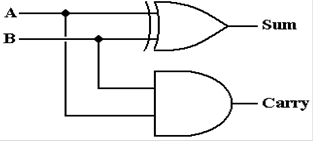An equivalent implementation is shown in order to facilitate discussion of the full–adder.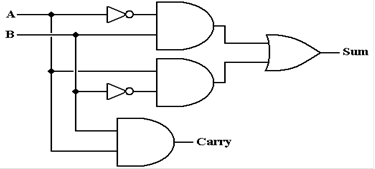The full–adder might be seen as equivalent to the tens or hundreds column in standard addition;
there must be provision for a carry–in from the column to the right.  Here again is the truth table

 A B Cin Sum Cout Result   = 2 · Cout + 1 · Sum 0 0 0 0 0 0 = 2 · 0 + 1 · 0 0 0 1 1 0 1 = 2 · 0 + 1 · 1 0 1 0 1 0 1 = 2 · 0 + 1 · 1 0 1 1 0 1 2 = 2 · 1 + 1 · 0 1 0 0 1 0 1 = 2 · 0 + 1 · 1 1 0 1 0 1 2 = 2 · 1 + 1 · 0 1 1 0 0 1 2 = 2 · 1 + 1 · 0 1 1 1 1 1 3 = 2 · 1 + 1 · 1

A bit of Boolean algebra will show that the Sum and Carry (out) can be represented as: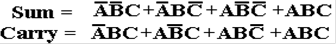A bit more Boolean algebra will result in the following simplification of the second expression.Here is the circuit implementing these two expressions.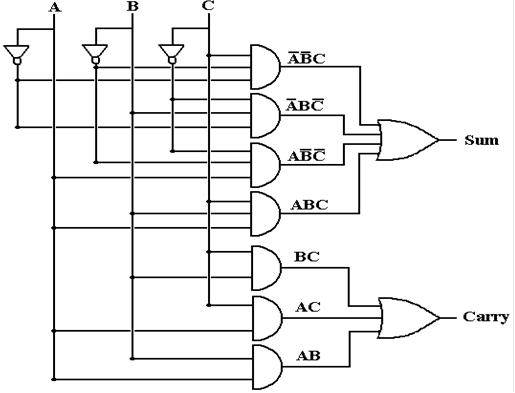This is the standard implementation of a full–adder.  There is another worth mention.

This second implementation of a full–adder appears in the text by Rob Williams.  It uses two
half–adders and an OR gate.  Here is the circuit.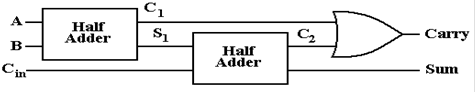First, we must show that the circuit does indeed function as a full–adder.  This is done
using a truth table.  Note a column for each intermediate result.  This is a full–adder.

 A B Cin C1 S1 C2 Carry Sum 0 0 0 0 0 0 0 0 0 0 1 0 0 0 0 1 0 1 0 0 1 0 0 1 0 1 1 0 1 1 1 0 1 0 0 0 1 0 0 1 1 0 1 0 1 1 1 0 1 1 0 1 0 0 1 0 1 1 1 1 0 0 1 1

A simple way to confirm that this is the truth table of a full–adder is to count the number of
1’s in the triple (A, B, Cin).  If the count is odd (1 or 3) the sum is 1, otherwise the sum is 0.
If the count is 2 or more, the carry is 1, otherwise the carry is 0.

We have just considered a full adder for two one–bit numbers with a carry–in (from somewhere).
We now extend the idea to multiple bit binary numbers.  Typical examples include 8, 16, 32, and
64 bit numbers.  Our example will focus on four bit numbers.  We add two four bit numbers
(A3, A2, A1, A0) and (B3, B2, B1, B0).

We begin with the block diagram of a full–adder.  Following standard practice in the study of
computer organization and architecture, once the inner details of a circuit have been mastered,
we no longer show them, but show the circuit as a single block.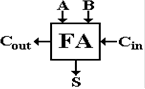We now extend this design to a four–bit parallel adder.  This design is the logical basis for the
adder in a modern ALU.  It is too slow for actual use.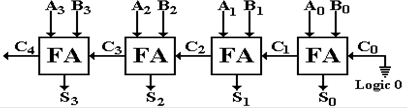Recall that the “units bit” addition with A0 and B0 could have been implemented as a
half–adder.  For reasons that will appear shortly, this was implemented as a full–adder
with the carry input tied to logic 0, here 0 volts (represented by the ground symbol).

This is called a “ripple carry” adder in that the carry bit ripples from right to left.  Each
full–adder has a time delay before the carry–out bit is valid.  Consider FA1, the one with inputs
A1, B1, and C1.  The input C1 does not become valid until some time after the inputs to FA0,
A0 and B0 become valid.  Another delay ensues before the outputs S1 and C1 are valid.

For details on the timing of a full–adder and a ripple carry adder, please refer to this author’s
textbook on Computer Architecture.

Binary Subtraction

We shall use a full–adder to perform binary subtraction by following the standard rule from
arithmetic: A – B = A + (–B).  We just need to negate one of the inputs to the full–adder and
we have a subtractor.  How do we do that?

All modern Arithmetic Logic Units implement integer arithmetic using the two’s–complement
form.  Thus we need to build a two’s–complement negator.  As an aside, we note that it is
possible to build an adder/subtractor for other formats, but that these designs are quite complex.
It is the simplicity of the standard two’s–complement unit that lead to its being the standard.

Remember the rule for negating an integer in two’s–complement arithmetic: take the
one’s–complement and add one.  As an expression, this is as follows: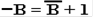But recall that taking the one’s–complement of a binary number is exactly the same as taking
the logical NOT of each of its bits.  Here is the case for the 4–bit number B3B2B1B0.  We begin
with a circuit to produce the one’s–complement of the number.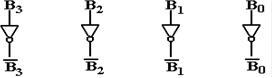In order to get the negative of the number, all we need to do is add 1 to it.  Of the several ways
this could be done, the best way is to set the carry–in of the units full–adder to 1.Consider the following positive 4–bit number.  B =  0100
Take the one’s complement to get this number
1011
Add one to the number to get the true negative
1100

If the left input to each FA had been the bit values of A, we would have (A – B).  Now we
try to convert this into a unit that will add or subtract.

In order to build the unit, we need a circuit element that can generate either the plain version
of its input or the one’s–complement of its input.  But we have exactly that in the XOR gate.
Here is the truth table for the XOR circuit, with two inputs: B and S (select).

 B S B Å S 0 0 0 1 0 1 0 1 1 1 1 0

Given the above, here is the full circuit for the 4–bit adder/subtractor.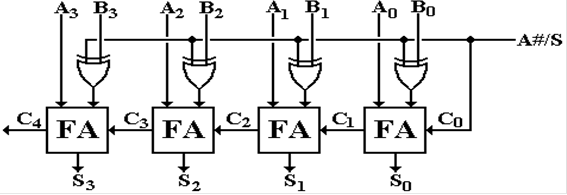Consider the case when A#/S = 0.  This indicates that addition is to take place.  This signal
is fed into the exclusive OR gates feeding the right inputs of the adders, passing the plain form
of the B input.  This feeds the units carry–in; the result is A + B + 0, or just A + B.

Now consider the case when A#/S = 1.  This indicates that subtraction is to take place.  This
is fed into the exclusive OR gates, passing the one’s complement of B into the right inputs of
the adder.  The signal feeds the units carry–in, thus the result we get is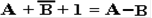.

Consider the following example of addition and subtraction.  In decimal, the numbers are
A = 5 and B = 2.  It should be obvious that A + B = 7 and A – B = 3.

Let’s do the arithmetic in 4–bit binary.  The addition is straightforward.

A         = 0101
B         =
0010
Sum     =
0111

In order to evaluate A – B, it is necessary first to take the two’s–complement of B.

B                                 = 0010
1’s complement           =
1101
1110

Here then is the addition to get A – B
A
0101      In the 4’s column, 1 + 1 with carry–in = 0 is 0, with carry–out = 1.
–B
1110      In the 8’s column, 1 + 0 with carry–in = 1 is 0, with carry–out = 1

0011      The carry out from the 8’s column is discarded.

Here is the circuit above when addition is called for; A#/S = 0.  Note that the selector input
to the exclusive OR function is a 0, so that the B values are passed unchanged.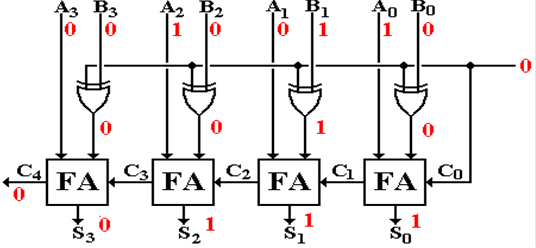Here is the circuit above when subtraction is called for; A#/S = 1.  The selector input to
the exclusive OR function is a 1, so that the value passed is the one’s–complement of B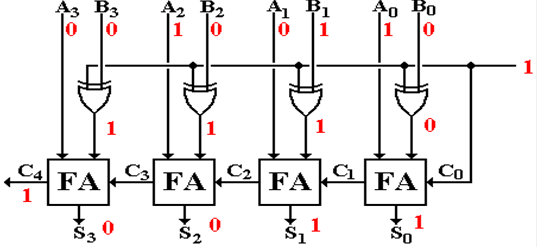As indicated above, these circuits are correct logical models of an adder/subtractor, but
they are not actually used.  Consider a 32–bit version of this ripple carry adder.  It would
have 32 full adders, connected in the same manner as above.

Such a ripple carry adder is much too slow for commercial use.  Each full–adder must wait on
the completion of the carry–out calculations of all the full–adders to its right before it can
begin calculating the sum and carry–out.

The full–adder for the sign bit (FA 31) must wait for all 31 full–adders (FA 0 to FA 30) to
its right to complete their calculations before starting.  That is a long wait.

The Shifter

Bits in an addressable unit, such as a byte or word, are considered to have position.  A shifter
shifts each bit in the unit by a fixed amount, with variations depending on type.

In our examples of shifting, we shall consider 8–bit bytes.  The term “byte”, coined by IBM, and
long thought to be trademarked by them, was chosen for the unit of storage appropriate to store
the binary coding for a character.  At the time, the complete IBM character set required seven
bits to encode; this was extended to 8 bits out of habit.

Each byte, having eight bits, has its bits numbered from 0 through 7.  Here is the bit numbering
scheme used by all manufacturers except IBM.

 Bit # 7 6 5 4 3 2 1 0 Value 1 0 0 1 0 1 1 0

Bit 7 is the most significant bit; bit 0 is the least significant bit.  In hexadecimal notation, this
would be represented as 96.  The unsigned decimal value is 9
·16 + 6 = 150.

Here are some examples of simple logical shifts, in which a 0 is shifted into the vacated “spot”.

The original value in binary:                               1001 0110
The original value right shifted one place:
0100 1011
The original value right shifted two places        0010 0101

The original value in binary:                               1001 0110
The original value left shifted one place:
0010 1100
The original value left shifted two places          0101 1000

There is some interesting arithmetic for unsigned integers hidden in these shift operators.  It is
more easily noticed for smaller numbers.  Decimal 11 in binary is
0000 1011.

The original value in binary:                            0000 1011, decimal value = 11
The original value shifted right one place
0000 0101, decimal value = 5
The original value shifted right two places
0000 0010, decimal value = 2.

The original value in binary:                            0000 1011, decimal value = 11
The original value shifted left one place
0001 0110, decimal value = 22
The original value shifted left two places
0010 1100, decimal value = 44.

In other words, for unsigned binary integers, a single left shift is equivalent to multiplication by
two, and a single right shift is equivalent to division by two, with the remainder discarded.  For
signed binary integers, the situation is only slightly more complicated.  The shift operations are
much more time efficient than either integer multiplication or integer division.  For this reason,
one often sees shift operations substituted for multiplications and divisions by powers of two.

The hardware for the simplest shift units shifts only one place at a time.  To shift left by three
places requires three calls to the shift operator.  There are two types of shifters that are more
efficient and hence more complex.  The logarithmic shifter has a number of stages that is the
logarithm (base 2) of the number of bits; a shifter for 16 bits would have four stages (16 = 24),
and a shifter for 32 bits would have five stages (32 = 25).

The most efficient shifter is the barrel shifter; it has one stage only.

In general, there are two types of shifts – left shifts and right shifts.  These names correspond
roughly to the way in which we would illustrate these shifts by drawing diagrams.  Each of the
two shift types comes in three varieties: logical, circular, and arithmetic.

The basic definition of each shift type is in terms of shifting by one place.  We should note that
multiple shifts are easily defined; shifting by N places must be equivalent to N single shifts.  For
convenience in designing a barrel shifter, we normally think in terms of shifting by N = a power
of two, so that a shift by 13 places is a shift by 1 place, followed by a shift by 4 places, and then
a shift by 8 places, as 13 = 1 + 4 + 8.

We shall illustrate the shift types by considering them as applied to an eight-bit shift register,
with contents labeled as R7 R6 R5 R4 R3 R2 R1 R0.  We use 1001 0110 as an example.

Logical Shifts

Logical shifts just move bits in the indicated direction, padding out with 0’s.  Shifts can be by
any count, but shifts by more than the size of the register leave it all 0’s.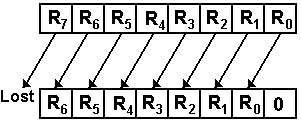For left shifting an N-bit register by 1 place
RJ+1
¬ RJ        for 0 £ J < (N – 1)
R0
¬ 0, R(N – 1) is lost

As an example of a shift of an 8-bit register
For a single left shift               1001 0110 becomes 0010 1100
Left shift by 2 places:             1001 0110 becomes 0101 1000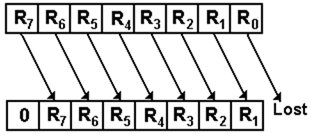For right shifts by 1 place
RJ+1
® RJ        for 0 £ J < (N – 1)
R(N – 1)
¬ 0, R0 is lost

As an example of a 8-bit register shift
For a single right shift 1001 0110 becomes 0100 1011

Right shift by 2 places:           1001 0110 becomes 0010 0101

Note that shifting either left or right by eight or more places produces the result 0000 0000,
so that the shift count will normally be in the range 0 through 7 inclusive.

The general rule for an N-bit register is that the shift count is usually in the range from
0 to (N – 1) inclusive, a modulo–N non–negative number.

Arithmetic Shifts

Arithmetic shifts are identical to logical shifts except that the sign bits are preserved.  Arithmetic
shifting is normally defined only for right shifts.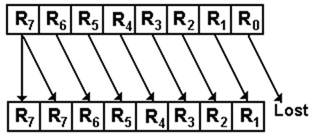For right shifts by 1 place
RJ+1
® RJ        for 0 £ J < (N – 1)
R(N – 1)
® R(N – 1), R0 is lost

As an example of an 8-bit register      1001 0110 becomes 1100 1011

The purpose of arithmetic shifts is to cause the right shift to become equivalent to division by
two on two’s-complement integers.  We use 8-bit two’s-complement arithmetic to illustrate the
correspondence of shifting to multiplication and division.  The range of this representation is
from – 128 to 127 inclusive.

Consider the number 52, represented as 0011 0100 in binary.  Taking the two’s-complement
of this binary pattern, we find that the representation of – 52 is 1100 1100.

We first apply successive arithmetic right shifts to both 52 and – 52.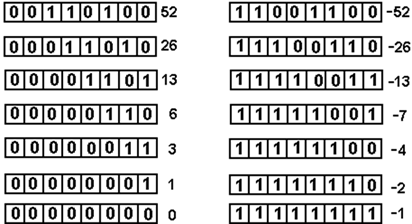We now apply successive logical left shifts to the same two numbers.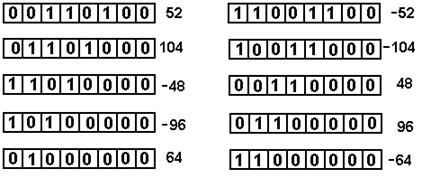Note that this corresponds to multiplication by two whenever the sign bit stays the same.

Circular Shifts

Circular shifts are identical to logical shifts except that bits “shifted off” one end are put at the other end, thus making the shift appear as a circle.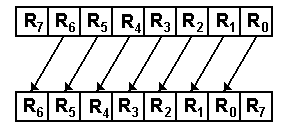For left shifts by 1 place
RJ+1
¬ RJ        for 0 £ J < (N – 1)       R0 ¬ R(N – 1), nothing is lost

As an example for an 8-bit shift
1001 0110 becomes 0010 1101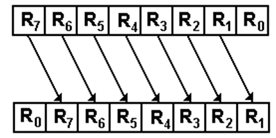For right shifts by 1 place
RJ+1
® RJ        for 0 £ J < (N – 1)
R(N – 1)
¬ R0

As an example of an 8-bit shift
1001 0110 becomes 0100 1011

The Barrel Shifter

To give a flavor of a barrel shifter, I design a single bit circular shifter for a 4–bit number.
We must begin with a table giving the output of the shifter in terms of the input R3R2R1R0.

 No shift Left Circular Right Circular Y3 R3 R2 R0 Y2 R2 R1 R3 Y1 R1 R0 R2 Y0 R0 R3 R1

Here is the circuit.  It is implemented with tri–state buffers.  When there is no shift, the red line
is asserted.  The green line is asserted for a single left shift and the blue line for a right shift.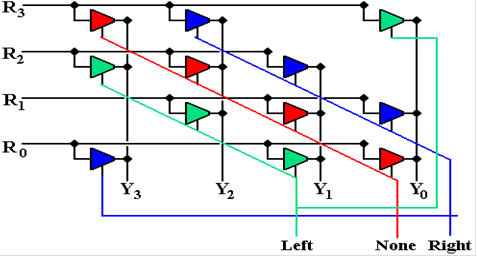As complex as the above circuit appears to be, it can grow much messier for reasonably sized
shift units.  The number of tri–state buffers scales quadratically as the number of bits in the
ALU; more specifically a shifter for an N–bit ALU would require 3N2 tri–states.  That would
be 768 tri–states for a 16–bit ALU and 3,072 tri–states for a 32–bit ALU.

In order to clarify the above circuit, we present the state of the circuit for each of the three shift
options: no shift, left shift by 1, and right shift by 1.  Note that for each diagram, each input line
is connected to precisely one output line.

First the no shift option.  The lines in red show the connections.  Other wires are not connected.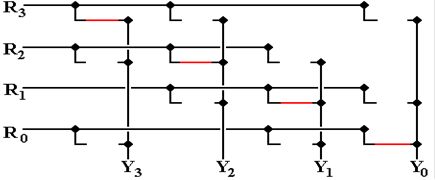Now the left shift option.  The lines in green show the connections.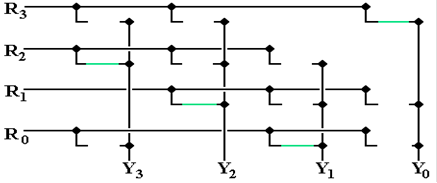And now the right shift option. The lines in blue show the connections.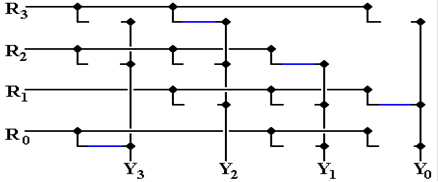Multiplication and Division

We note immediately that multiplication of two N–bit integers yields a product with 2N bits.  For
that reason, we shall discuss N–bit multiplication with a 2N–bit product, and N–bit division with
a 2N–bit dividend, an N–bit divisor, an N–bit quotient, and N–bit remainder.  This “doubling of
the digits” is seen in decimal as well as binary multiplication.

Decimal:          9,999 · 9,999  = 99,980,001
Binary             1111
· 1111    = 1110 0001    (15 · 15 = 225)

The most common implementations of multipliers call for two 16–bit numbers with a 32–bit
product, and two 32–bit numbers with a 64–bit product.

We begin with a consideration of multiplication for unsigned positive integers.  At one level, this
is quite simple, as the “times table” is very small.  Here it is.

 A B A·B 0 0 0 0 1 0 1 0 0 1 1 1

One might note that this is exactly the truth table for the logical AND function, which is denoted
by the same symbol as multiplication.  This might suggest the use of the logical AND gate in a
multiplier; the true circuits are even simpler.  Consider a labeled example.

1011                      the multiplicand, with decimal value 11

1001                      the multiplier, with decimal value 9

1011
0000
0000
the four partial products
1011___
1100011
the product, with decimal value 99

Note that there are four partial products, one for each bit in the multiplier.  Each partial product
is the length of the multiplicand, and is either a copy of the multiplicand or all 0.  The standard
assumption is that the multiplicand and multiplier have equal length, each having the length of
the standard integer in the architecture.  All commercial designs allow different lengths for
integer representations (8–bit, 16–bit, 32–bit, etc.), providing a number of distinct multiplication
operations (8–bit by 8–bit, 16–bit by–16 bit).

Modern multiplication algorithms are based on shifting and adding.  This allows one to use the
minimum number of registers required to hold the operands and the results.  The standard
approach calls for setting up a result of 2N bits, initialized to zero.  The multiplier is then
examined right to left.  If the bit is 1, the multiplicand is added to the result.  If not, nothing

The next step is to shift the multiplicand left by one, and consider the next bit in the
multiplier.  We illustrate this algorithm with two 4–bit numbers and an 8–bit product.

For this example, label the multiplier bits as M3M2M1M0; M3 = 0, M2 = 1, M1 = 1, M0 = 1.
For 4–bit multiplication, we initialize the register set used for the product to eight 0’s.

At the start, the situation is as follows.   Multiplicand         1011
Results
00000000

M0 = 1, add multiplicand to results         Multiplicand         1011
Results
00001011

Shift the results register set right             Multiplicand         1011
Results
00001011

M1 = 1, add multiplicand to results         Multiplicand         1011
Results
00100001

Shift the results register set right             Multiplicand         1011
Results
00100001

M2 = 1, add multiplicand to results         Multiplicand         1011
Results
01001101

Shift the results register set right             Multiplicand         1011
Results
01001101

M3 = 0, do not add.                                 Multiplicand         1011
Results
01001101

Division

Standard division follows the same strategy as classical long division, except that it cannot
use any human–style inspection to compare two numbers.  The only way for an ALU to
compare two numbers is to perform a subtraction and test the sign of the result.  Here we shall
describe a variant of division called “restoring division”, by which we mean that a subtraction
will be performed and, if the result is negative, the original value will be restored by an addition.

Consider the manual algorithm as applied to unsigned binary division.  We shall apply long division
to apply the divisor 1011 (decimal 11) to the dividend 10010011 (decimal 147).  In the manual algorithm,
we place the divisor immediately below the dividend, test if it is too large, and proceed accordingly.

_________
1011 )10010011
1011

At this point, a human would note that the divisor is larger than the 4–bit part of the dividend
immediately above it and move on.  The ALU will perform the subtraction, get
1110 (or
minus 2), then add back the
1011 to get 1001, and only then move on.

Now the five–bit part of the dividend, 10010, is compared to the four–bit divisor, 1011, and
subtracted from it.  A “
1” is written directly above the units column for the divisor.

_00001___
1011 )10010011
1011
0111

Next a 0 is “brought down”, the divisor shifted once more to the right, and compared.  The
divisor is smaller than the partial remainder.  The subtraction is performed.

_000011__
1011 )10010011
1011
01110
1011
0011

We now finish the division using the standard manual practice.

_00001101
1011 )10010011
1011
01110
1011
001111
1011
100